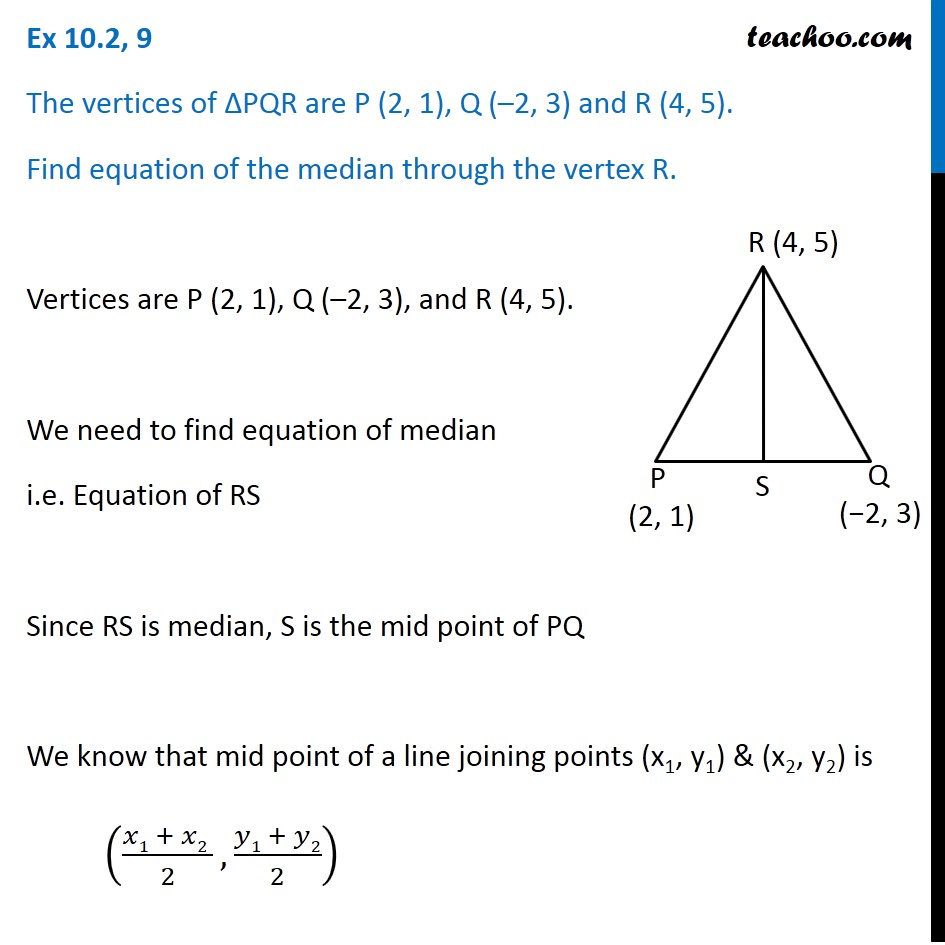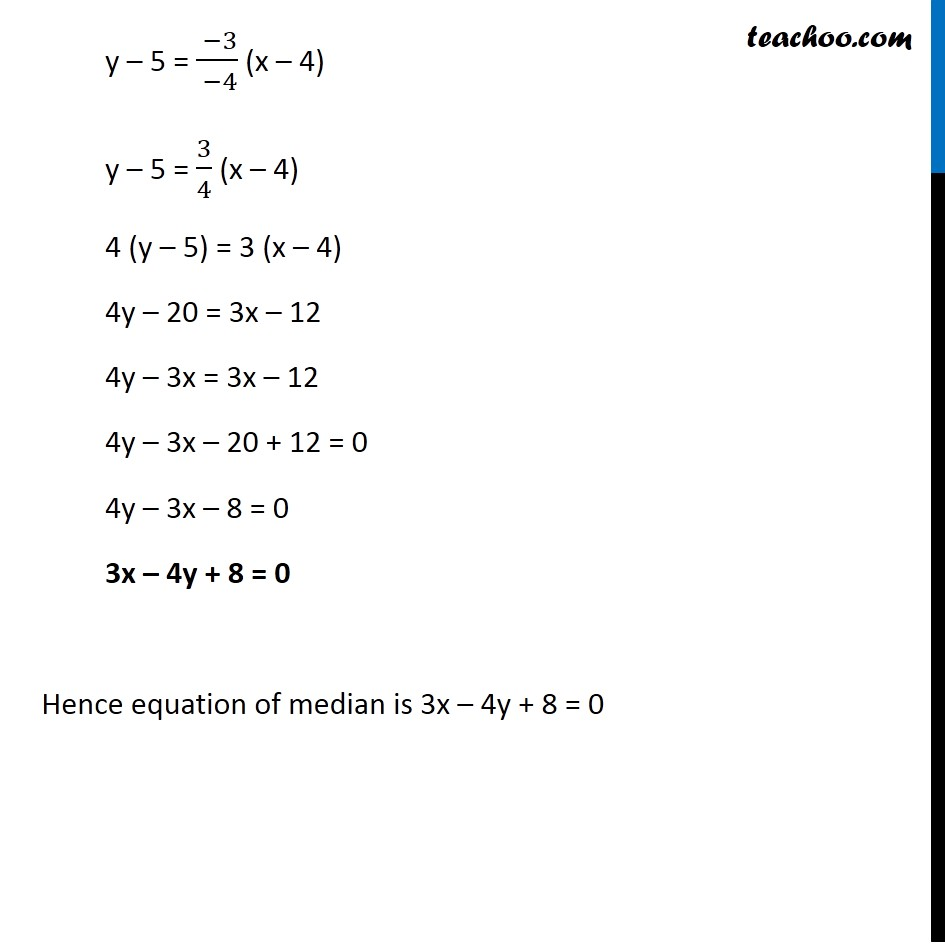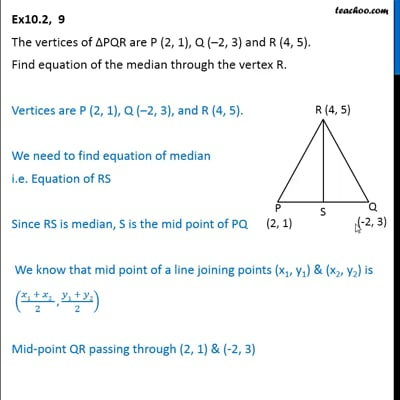Ex 10.2

Chapter 10 Class 11 Straight Lines (Term 1)
Serial order wiseThis video is only available for Teachoo black users

### Transcript

Ex 10.2, 9 The vertices of ΔPQR are P (2, 1), Q (–2, 3) and R (4, 5). Find equation of the median through the vertex R. Vertices are P (2, 1), Q (–2, 3), and R (4, 5). We need to find equation of median i.e. Equation of RS Since RS is median, S is the mid point of PQ We know that mid point of a line joining points (x1, y1) & (x2, y2) is ((𝑥1 + 𝑥2 )/2, (𝑦1 + 𝑦2)/2) Mid-point QR passing through (2, 1) & (−2, 3) S = ((2 + ( −2))/2,(1 + 3)/2) S = ((2 − 2)/2,4/2) S = (0, 2) Now we need to find equation of line passing through R (4, 5) & S (0, 2) We know that equation of line through two points (x1, y1) & (x2, y2) is y – y1 = (𝑦_2 − 𝑦_1)/(𝑥_2 − 𝑥_1 ) (x – x1) Putting values (y – 5) = (2 − 5)/(0 − 4) (x – 4) y – 5 = ( −3)/( −4) (x – 4) y – 5 = 3/4 (x – 4) 4 (y – 5) = 3 (x – 4) 4y – 20 = 3x – 12 4y – 3x = 3x – 12 4y – 3x – 20 + 12 = 0 4y – 3x – 8 = 0 3x – 4y + 8 = 0 Hence equation of median is 3x – 4y + 8 = 0